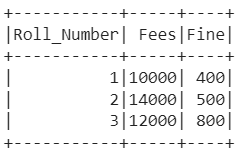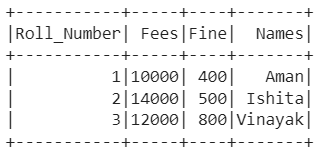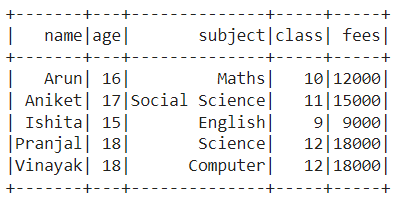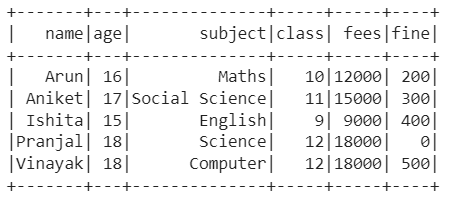Open in App
Not now

# Adding a Column in Dataframe from a list of values using a UDF Pyspark

• Last Updated : 23 Jan, 2023

In this article, we are going to learn how to add a column from a list of values using a UDF using Pyspark in Python.

A data frame that is similar to a relational table in Spark SQL, and can be created using various functions in SparkSession is known as a Pyspark data frame. There occur various circumstances in which we get data in the list format but you need it in the form of a column in the data frame. If a similar situation has occurred with you, then you can do it easily by assigning increasing IDs to the data frame and then adding the values in a column. Read the article further to know more about it in detail.

### Steps to  add a column from a list of values using a UDF

Step 1: First of all, import the required libraries, i.e., SparkSession, functions, IntegerType, StringType, row_number, monotonically_increasing_id, and Window. The SparkSession is used to create the session, while the functions give us the authority to use the various functions available in Pyspark. The IntegerType and StringType are used to create a new integer or string column respectively. The row_number is used to return a sequential number starting from 1 within a window partition, while monotonically_increasing_id is used to generate monotonically increasing 64-bit integers. The Window is used to operate on a group of rows and return a single value for every input row.

```from pyspark.sql import SparkSession, functions as F
from pyspark.sql.types import IntegerType, StringType
from pyspark.sql.functions import row_number, monotonically_increasing_id
from pyspark.sql.window import Window```

Step 2: Now, create a spark session using the getOrCreate() function.

`spark_session = SparkSession.builder.getOrCreate()`

Step 3: Then, create a data frame using createDataFrame() or read the CSV file.

```data_frame=csv_file = spark_session.read.csv('#Path of CSV file',
sep = ',', inferSchema = True,

or

```data_frame=spark_session.createDataFrame([(column_1_data), (column_2_data), (column_3_data)],
['column_name_1', 'column_name_2', 'column_name_3'])```

Step 4: Later on, define a list that needs to be added as a column to the data frame.

`list_data = [list_value_1, list_value_2, list_value_3 ]`

Step 5: Moreover, create a column having continuously increasing IDs using monotonically_increasing_id for the data frame according to which the list can be added to the data frame column.

`df = df.withColumn("num_id", row_number().over(Window.orderBy(monotonically_increasing_id())))`

Step 6: Further, define a user-defined function, i.e., UDF with column values and column type as arguments. As the index value starts from 0, thus we assign data according to the row index by subtracting the row number from 1.

`labels_udf = F.udf(lambda indx: fine_data[indx-1] , IntegerType())`

Step 7: Later on, create a column by calling the user-defined function and assigning the values.

`new_df = df.withColumn('new_column_name', labels_udf('num_id'))`

Step 8: Finally, drop the increasing Id column and display the data frame.

`new_df.drop('num_id').show()`

### Example 1:

In the example, we have created a data frame with three columns ‘Roll_Number‘, ‘Fees‘, and ‘Fine‘ as follows:Once created, we assigned continuously increasing IDs to the data frame using the monotonically_increasing_id() function. Also, we defined a list of values, i.e., student_names which need to be added as a column to a data frame. Then, with the UDF increasing Id’s, we assigned values of the list as a column to the data frame and finally displayed the data frame after dropping the increasing Id’s column.

## Python3

 `# PySpark - Adding a Column from a list of values using a UDF ` ` `  `# Import the libraries SparkSession, functions, IntegerType,  ` `# StringType, row_number, monotonically_increasing_id and Window ` `from` `pyspark.sql ``import` `SparkSession, functions as F ` `from` `pyspark.sql.types ``import` `IntegerType, StringType ` `from` `pyspark.sql.functions ``import` `row_number, monotonically_increasing_id ` `from` `pyspark.sql.window ``import` `Window ` ` `  `# Create a spark session using getOrCreate() function ` `spark_session ``=` `SparkSession.builder.getOrCreate() ` ` `  `# Create a user defined function to assign student names  ` `# according to the row index by subtracting row number from 1 ` `labels_udf ``=` `F.udf( ` `  ``lambda` `indx: student_names[indx``-``1``], ` `                   ``StringType()) ` ` `  `# Create a data frame with three columns 'Roll_Number,' 'Fees' and 'Fine' ` `df``=``spark_session.createDataFrame( ` `  ``[(``1``, ``10000``, ``400``), (``2``, ``14000` `, ``500``), ` `                    ``(``3``, ``12000` `, ``800``)], ` `  ``[``'Roll_Number'``, ``'Fees'``, ``'Fine'``]) ` ` `  `# Define a list of elements ` `student_names ``=` `[``'Aman'``,``'Ishita'``,``'Vinayak'``] ` ` `  `# Create a column with continuous increasing Id's ` `df ``=` `df.withColumn( ` `  ``"num_id"``, row_number().over(Window.orderBy( ` `              ``monotonically_increasing_id()))) ` ` `  `# Create a new column by calling the user defined function ` `new_df ``=` `df.withColumn(``'Names'``, ` `                       ``labels_udf(``'num_id'``)) ` ` `  `# Delete the increasing Id's column and display the data frame ` `new_df.drop(``'num_id'``).show()`

Output:### Example 2:

In this example, we have used a data set (link), i.e., basically, a 5*5 data set as follows:Then, we assigned continuously increasing IDs to the data frame using the monotonically increasing_id function. Also, we defined a list of values, i.e., fine_data which needs to be added as a column to the data frame. Then, with the UDF increasing Id’s, we assigned values of the list as a column to the data frame and finally displayed the data frame after dropping the increasing Id’s column.

## Python3

 `# PySpark - Adding a Column from a list of values using a UDF ` ` `  `# Import the libraries SparkSession, functions, IntegerType,  ` `# StringType, row_number, monotonically_increasing_id and Window ` `from` `pyspark.sql ``import` `SparkSession, functions as F ` `from` `pyspark.sql.types ``import` `IntegerType, StringType ` `from` `pyspark.sql.functions ``import` `row_number, monotonically_increasing_id ` `from` `pyspark.sql.window ``import` `Window ` ` `  `# Create a spark session using getOrCreate() function ` `spark_session ``=` `SparkSession.builder.getOrCreate() ` ` `  `# Create a user defined function to assign student names  ` `# according to the row index by subtracting row number from 1 ` `labels_udf ``=` `F.udf( ` `  ``lambda` `indx: fine_data[indx``-``1``], ` `  ``IntegerType()) ` ` `  `# Read the CSV file ` `df``=``csv_file ``=` `spark_session.read.csv(``'/content/student_data.csv'``, ` `     ``sep ``=` `','``, inferSchema ``=` `True``, header ``=` `True``) ` ` `  `# Define a list of elements ` `fine_data ``=` `[``200``,``300``,``400``,``0``,``500``] ` ` `  `# Create a column with continuous increasing Id's ` `df ``=` `df.withColumn( ` `  ``"num_id"``, row_number().over( ` `  ``Window.orderBy(monotonically_increasing_id()))) ` ` `  `# Create a new column by calling the user defined function ` `new_df ``=` `df.withColumn(``'fine'``, ` `                       ``labels_udf(``'num_id'``)) ` ` `  `# Delete the increasing Id's column and display the data frame ` `new_df.drop(``'num_id'``).show()`

Output:My Personal Notes arrow_drop_up
Related Articles